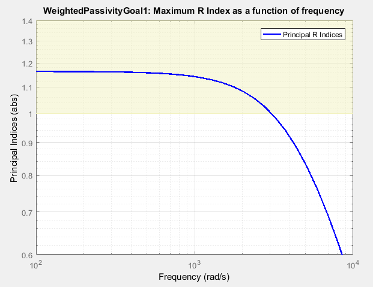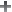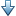## Weighted Passivity Goal

### Purpose

Enforce passivity of a frequency-weighted transfer function when tuning in Control System Tuner.

### Description

Weighted Passivity Goal enforces the passivity of H(s) = WL(s)T(s)WR(s), where T(s) is the transfer function from specified inputs to outputs. WL(s) and WR(s) are frequency weights used to emphasize particular frequency bands. A system is passive if all its I/O trajectories (u(t),y(t)) satisfy:

`${\int }_{0}^{T}y{\left(t\right)}^{T}u\left(t\right)dt>0,$`

for all T > 0. Weighted Passivity Goal creates a constraint that enforces:

`${\int }_{0}^{T}y{\left(t\right)}^{T}u\left(t\right)dt>\nu {\int }_{0}^{T}u{\left(t\right)}^{T}u\left(t\right)dt+\rho {\int }_{0}^{T}y{\left(t\right)}^{T}y\left(t\right)dt,$`

for the trajectories of the weighted transfer function H(s), for all T > 0. To enforce the overall passivity condition, set the minimum input passivity index (ν) and the minimum output passivity index (ρ) to zero. To enforce an excess of passivity at the inputs or outputs of the weighted transfer function, set ν or ρ to a positive value. To permit a shortage of passivity, set ν or ρ to a negative value. See `getPassiveIndex` for more information about these indices.In Control System Tuner, the shaded area on the plot represents the region in the frequency domain in which the tuning goal is not met. The plot shows the value of the index described in Algorithms.

#### Creation

In the Tuning tab of Control System Tuner, select New Goal > Weighted Passivity Goal.

#### Command-Line Equivalent

When tuning control systems at the command line, use `TuningGoal.WeightedPassivity` to specify a step response goal.

### I/O Transfer Selection

Use this section of the dialog box to specify the inputs and outputs of the transfer function that the tuning goal constrains. Also specify any locations at which to open loops for evaluating the tuning goal.

• Specify input signals

Select one or more signal locations in your model as inputs to the transfer function that the tuning goal constrains. To constrain a SISO response, select a single-valued input signal. For example, to constrain the gain from a location named `'u'` to a location named `'y'`, clickAdd signal to list and select `'u'`. To constrain the passivity of a MIMO response, select multiple signals or a vector-valued signal.

• Specify output signals

Select one or more signal locations in your model as outputs of the transfer function that the tuning goal constrains. To constrain a SISO response, select a single-valued output signal. For example, to constrain the gain from a location named `'u'` to a location named `'y'`, clickAdd signal to list and select `'y'`. To constrain the passivity of a MIMO response, select multiple signals or a vector-valued signal.

• Compute input/output gain with the following loops open

Select one or more signal locations in your model at which to open a feedback loop for the purpose of evaluating this tuning goal. The tuning goal is evaluated against the open-loop configuration created by opening feedback loops at the locations you identify. For example, to evaluate the tuning goal with an opening at a location named `'x'`, clickAdd signal to list and select `'x'`.

Tip

To highlight any selected signal in the Simulink® model, click. To remove a signal from the input or output list, click. When you have selected multiple signals, you can reorder them usingand. For more information on how to specify signal locations for a tuning goal, see Specify Goals for Interactive Tuning.

### Weights

Use the Left weight WL and Right weight WR text boxes to specify the frequency-weighting functions for the tuning goal. H(s) = WL(s)T(s)WR(s), where T(s) is the transfer function from specified inputs to outputs.

WL provides the weighting for the output channels of H(s), and WR provides the weighting for the input channels. You can specify scalar weights or frequency-dependent weighting. To specify a frequency-dependent weighting, use a numeric LTI model whose magnitude represents the desired weighting function. For example, enter `tf(1,[1 0.01])` to specify a high weight at low frequencies that rolls off above 0.01 rad/s.

If the tuning goal constrains a MIMO transfer function, scalar or SISO weighting functions automatically expand to any input or output dimension. You can specify different weights for each channel by specifying matrices or MIMO weighting functions. The dimensions H(s) must be commensurate with the dimensions of WL and WR. For example, if the constrained transfer function has two inputs, you can specify `diag([1 10])` as WR.

If you are tuning in discrete time, you can specify the weighting functions as discrete-time models with the same sampling time as you use for tuning. If you specify the weighting functions in continuous time, the tuning software discretizes them. Specifying the weighting functions in discrete time gives you more control over the weighting functions near the Nyquist frequency.

### Options

Use this section of the dialog box to specify additional characteristics of the step response goal.

• Minimum input passivity index

Enter the target value of ν in the text box. To enforce an excess of passivity at the specified inputs, set ν > 0. To permit a shortage of passivity, set ν < 0. By default, the passivity goal enforces ν = 0, passive at the inputs with no required excess of passivity.

• Minimum output passivity index

Enter the target value of ρ in the text box. To enforce an excess of passivity at the specified outputs, set ρ > 0. To permit a shortage of passivity, set ρ < 0. By default, the passivity goal enforces ρ = 0, passive at the outputs with no required excess of passivity.

• Enforce goal in frequency range

Limit the enforcement of the tuning goal to a particular frequency band. Specify the frequency band as a row vector of the form `[min,max]`, expressed in frequency units of your model. For example, to create a tuning goal that applies only between 1 and 100 rad/s, enter `[1,100]`. By default, the tuning goal applies at all frequencies for continuous time, and up to the Nyquist frequency for discrete time.

• Apply goal to

Use this option when tuning multiple models at once, such as an array of models obtained by linearizing a Simulink model at different operating points or block-parameter values. By default, active tuning goals are enforced for all models. To enforce a tuning requirement for a subset of models in an array, select Only Models. Then, enter the array indices of the models for which the goal is enforced. For example, suppose you want to apply the tuning goal to the second, third, and fourth models in a model array. To restrict enforcement of the requirement, enter `2:4` in the Only Models text box.

### Algorithms

When you tune a control system, the software converts each tuning goal into a normalized scalar value f(x). Here, x is the vector of free (tunable) parameters in the control system. The software then adjusts the parameter values to minimize f(x) or to drive f(x) below 1 if the tuning goal is a hard constraint.

For Weighted Passivity Goal, for a closed-loop transfer function T(s,x) from the specified inputs to the specified outputs, and the weighted transfer function H(s,x) = WL(s)T(s,x)WR(s), f(x) is given by:

`$f\left(x\right)=\frac{R}{1+R/{R}_{\mathrm{max}}},\text{ }{R}_{\mathrm{max}}={10}^{6}.$`

R is the relative sector index (see `getSectorIndex`) of [H(s,x); I], for the sector represented by:

`$Q=\left(\begin{array}{cc}2\rho & -I\\ -I& 2\nu \end{array}\right),$`

where ρ is the minimum output passivity index and ν is the minimum input passivity index specified in the dialog box. Rmax is fixed at 106, included to avoid numeric errors for very large R.

This tuning goal imposes an implicit minimum-phase constraint on the weighted transfer function H + I. The transmission zeros of H + I are the stabilized dynamics for this tuning goal. The Minimum decay rate and Maximum natural frequency tuning options control the lower and upper bounds on these implicitly constrained dynamics. If the optimization fails to meet the default bounds, or if the default bounds conflict with other requirements, on the Tuning tab, use Tuning Options to change the defaults.